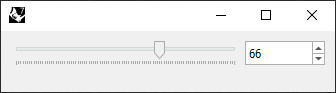# Synchronize values in Eto forms

I’m working on a custom Eto form with GhPython and looking to synchronize values from both controls, in this case a slider and a numeric updownWhen slider value is changed, the numeric should update as well as the oposite, when the numeric value changes, the slider updates too, keeping them syncronized.
So far I’ve created the controls but not sure how to syscronize both, does anyone knows how to achieve this?
Here’s the code

``````import Rhino
import System
import Rhino.UI
import Eto.Drawing as drawing
import Eto.Forms as forms

class OptionsPanel(forms.Form):

def __init__(self):
self.Size = drawing.Size(350,100)
self.m_slider = forms.Slider()
self.m_slider.MinValue = 1
self.m_slider.MaxValue = 100
self.m_slider.Value = 1
self.m_slider.ValueChanged += self.OnSliderValueChanged
self.m_numeric_updown = forms.NumericUpDown()
self.m_numeric_updown.DecimalPlaces = 0
self.m_numeric_updown.Increment = 1
self.m_numeric_updown.MaxValue = 100.0
self.m_numeric_updown.MinValue = 1.0
self.m_numeric_updown.Value = 1
self.m_numeric_updown.ValueChanged += self.OnSliderValueChanged

layout = forms.DynamicLayout()
layout.Spacing = drawing.Size(5, 5)
self.Content = layout

def OnSliderValueChanged(self, sender, e):
ghenv.Component.ExpireSolution(True)

def GetNumber1(self):
return self.m_slider.Value

def GetNumber2(self):
return self.m_numeric_updown.Value

if show == True:
if 'form' in globals():
form.Close()
form = OptionsPanel();
form.Show()

if 'form' in globals():
value1 = form.GetNumber1()
value2 = form.GetNumber2()
``````

Each control should have its own function ‘OnXXXValueChanged’. When one function is called due to change of one control, set the value on the other control and vice versa.

Check this code

Thanks @seghierkhaled
Actually looking into that code helped also to learn other stuff, very interesting to explore the possibilities available on these forms.
Another question off-topic, do you know how to load it on the center of Rhino viewport?
I’ve looked for it but just found the position based on the screen, not on Rhino itself.

Hi @mats_magnunson, see this example which works without event bubbling.

_
c.

2 Likes

That’s a far more elegant solution indeed! Thanks

1 Like

This is very good, thank you for sharing that line of code.